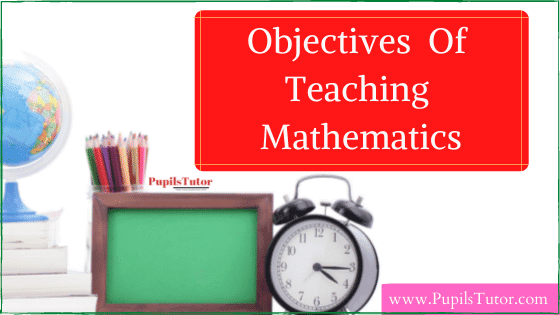What Is The Main Focus Of Mathematics Education? | Objectives Of Teaching MathematicsWrite the instructional Objectives for a mathematics lesson.

Some of the common objectives of teaching mathematics are:

1. Teaching fundamental numeracy skills to all students.
2. Teaching heuristics and other problem-solving strategies to solve non-routine problems.
3. Teaching selected areas of mathematics such as:
• Teaching Euclidean geometry as an example of an 'axiomatic system' and a 'model of deductive reasoning'.
• Teaching Calculus is an example of the 'intellectual achievements' of the modern world.
4. Teaching abstract mathematical concepts at an early age such as
• Set,
• Function etc.
5. Teaching mathematics to students for future livelihood. For instance,
• Teaching 'practical mathematics' to prepare students to follow a trade or craft.
• Teaching 'advanced mathematics' to students who wish to follow a career in fields like
• Engineering,
• Science and Technology,
• Mathematics etc.

B.Ed

B.Ed Notes

Pedagogy of Mathematics

Teaching of Mathematics

Tags:

What Is The Main Focus Of Mathematics Education? Notes

Some Specific Objectives, Purpose Of Teaching Mathematics Notes For B.Ed In English Medium

What Is Mathematics In Education - What Does Mathematics Focus On? Notes And Study Material, PDF, PPT, Assignment For B.Ed 1st and 2nd Year, DELED, M.Ed, CTET, TET, Entrance Exam, All Teaching Exam Test Download Free For Pedagogy of Maths And Teaching of Mathematics Subject.

Important Questions For Exam:

Write about an objective of teaching maths?

Explain the objectives of teaching mathematics in schools.

Write the instructional Objectives for a mathematics lesson.

Check Also: